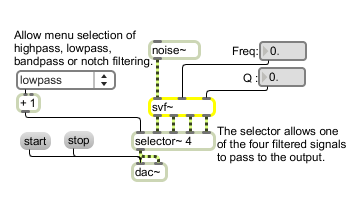# svf~

State-variable filter with simultaneous outputs

## Description

The svf~ object is an implementation of a state-variable filter algorithm described in Hal Chamberlin's book, "Musical Applications of Microprocessors." A unique feature of this filter object is that it produces lowpass, highpass, bandpass, and bandreject (notch) output simultaneously - all four are available as outlets.

## Arguments

Name Type Opt Description
initial-gain center-frequency and Q float opt Numbers set the initial gain, center frequency, and Q. The default values are 0 for gain, 0 for center frequency, and 0.01 for Q.
Hz symbol opt Sets the frequency input mode to Hz (the default mode - hence this is the same as providing no mode argument).
linear symbol opt Sets the frequency input mode to linear (0 -1).
radians symbol opt Sets the frequency input mode to radians (0 -1).

## Messages

 int center-frequency/Q [int] Converted to float. float center-frequency/Q [float] In middle and right inlets: A float can be sent in the two right inlets to change the center frequency and Q of the filter. By default, the center frequency is expressed in Hz, where the allowable range is from 0 to one fourth of the current sampling rate. For convenience, svf~ has two additional input modes that use the more conventional input range, 0 - 1. (see the linear and radians messages). If a signal is connected to one of the inlets, a number received in that inlet is ignored. The values are sampled once every signal vector. Hz In either inlet: Sets the frequency input mode to Hz (the default). clear Clears the object's sample-memory in case of a blow-up. linear In any inlet: Sets the frequency input mode to linear (0 - 1). Linear mode is simply a scaled version of the standard Hz mode, except that values in the 0-1 range traverse the full frequency range. radians In any inlet: Sets the frequency input mode to radians (0 - 1). Radians mode lets you set the center frequency directly -- while the input has the same range (0-1), the output has a curved frequency response that is closer to the exponential pitch scale of the human ear. signal In left inlet: Signal to be filtered. In middle inlet: Sets the filter center frequency in Hz. In right inlet: Sets the bandpass filter "Q" - roughly, the sharpness of the filter - where Q is defined as the filter bandwidth divided by the center frequency. Useful Q values are typically between 0.01 and 500.

## Output

signal: The filtered input signal.

## ExamplesFour filter outputs are simultaneously available from the svf~ object